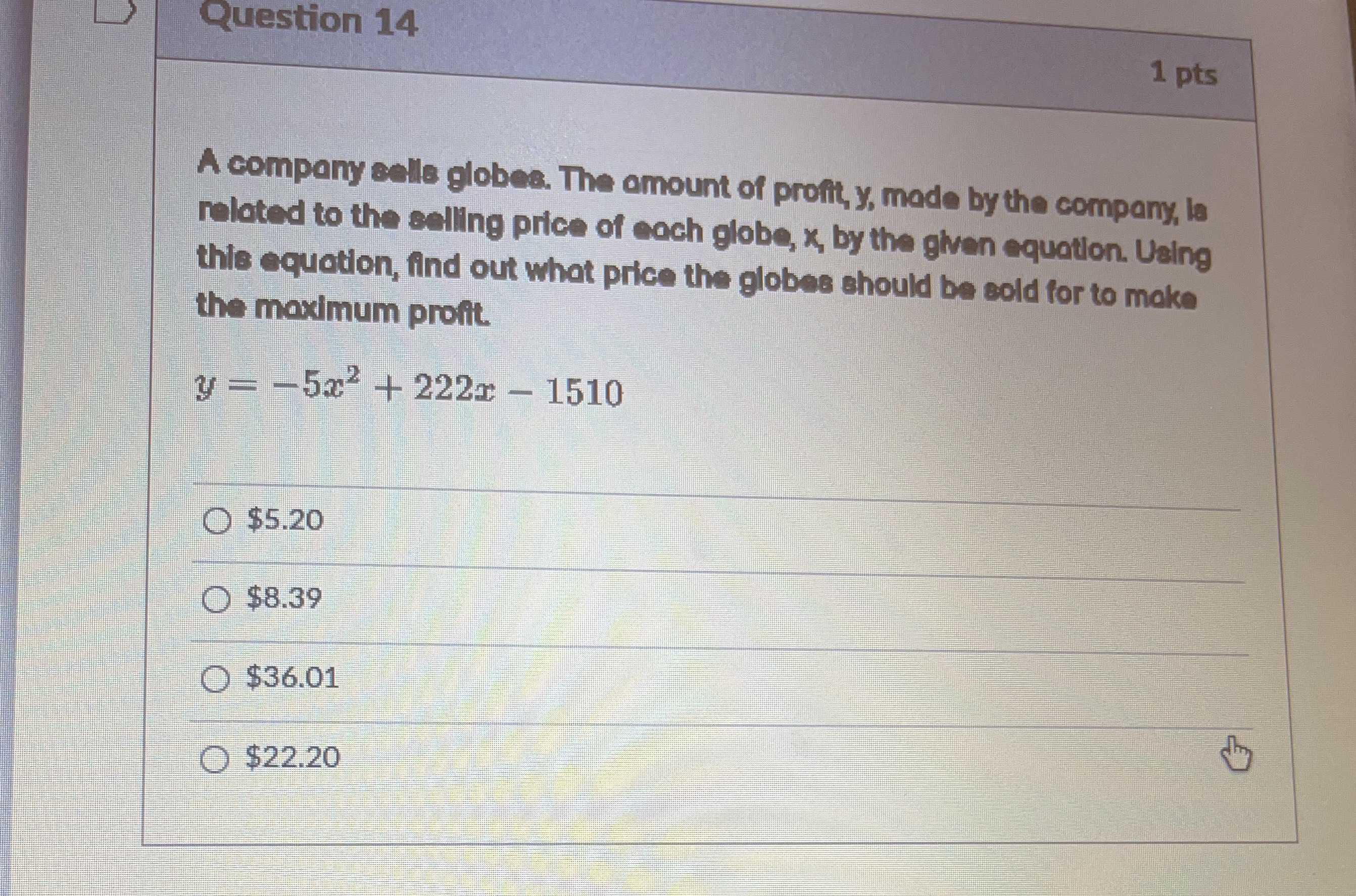### ¿Todavía tienes preguntas de matemáticas?

Pregunte a nuestros tutores expertos
Algebra
PreguntaA company ealle globee. The omount of proili, $$y$$ , made by the company, le reloted to the exlling price of each globe, $$x$$ , by the glven equation. Using the equotion, find out what price the globes should be eold for to mako the maximum proit.

$$y = - 5 x ^ { 2 } + 222 x - 1510$$

$$\ 5.20$$

$$\ 8.39$$

$$\ 36.01$$

$$\ 22.20$$